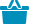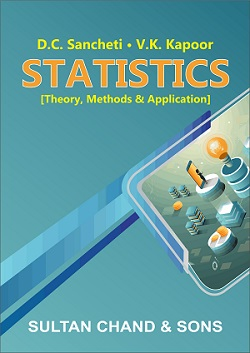Cart

# Statistics – Theory Methods & Applications#### Quick Overview

Authored By

Publisher: Sultan Chand & Sons

Publishing Year: 2010

Size (mm): 220.00 x 140.00

ISBN: 81-8054-612-9

Page nos.: xii + 1447

MRP: 695.00

Subject: Mathematical Sciences

₹695.00

Special Features

• A conscientious effort has been made all through to keep the treatment simple, lucid and non-mathematical.
• The theoretical description of each sub-section has been followed by simple illustrations. Complex problems have been solved thereafter.
• Exercises at the end of each chapter are substantially reorganised. Hints and answers have been provided for most of the exercises.
• Solved examples and unsolved problems have been drawn from examination papers of M.Com., B.Com., M.A. (Eco.), B.A. (Eco.) PE-I, I.C.W.A., etc.
• ‘Objective Type’ questions are also there at the end of each chapter.

• Meaning and Scope
• Statistical Survey
• Classification and Tabulation
• Diagrammatic and Graphic Presentation
• Measures of Averages
• Measures of Dispersion
• Skewness, Moments & Kurtosis
• Correlation Analysis
• Regression Analysis
• Index Numbers
• Analysis of Time Series
• Association of Attributes
• Interpolation
• Probability and Expected Value
• Statistical Decision Theory
• Theoretical Distributions
• Sampling and Designing of a Sample Survey
• Test of Hypothesis
• Chi-Square Test
• F-Test and Analysis of Variance
• Statistical Quality Control
• Multiple & Partial Correlation and Regression
• Vital Statistics
• Linear Programming

ISBN13: 978-81-8054-612-9

Weight: 1400.00

Edition: 7th Revised Edition

Language: English

Title Code: 555

#### Author

Authored By : Kapoor V.K., Sancheti D.C.